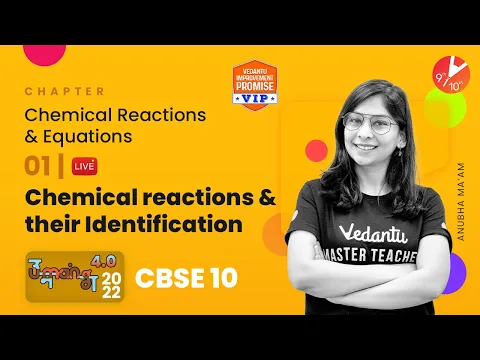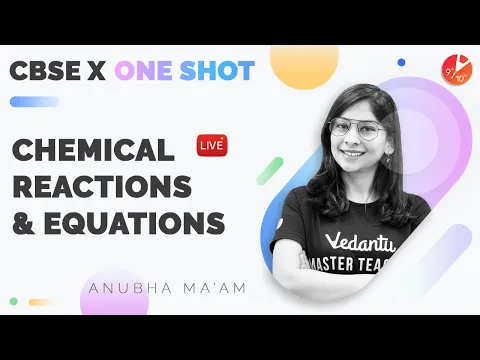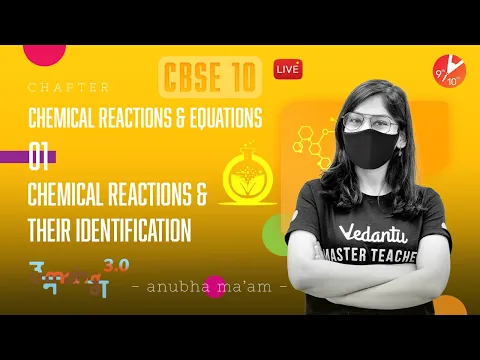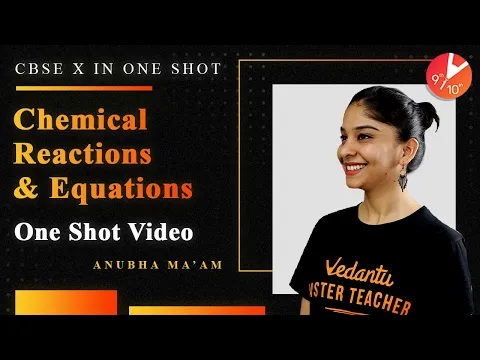Courses
Courses for Kids
Free study material
Free LIVE classes
More

# NCERT Solutions for Class 10 Science Chapter 1 Chemical Reactions and Equations in Hindi## NCERT Solutions for Class 10 Science Chapter 1 Chemical Reactions and Equations in Hindi PDF Download

Download the Class 10 Science NCERT Solutions in Hindi medium and English medium as well offered by the leading e-learning platform Vedantu. If you are a student of Class 10, you have reached the right platform. The NCERT Solutions for Class 10 Science in Hindi provided by us are designed in a simple, straightforward language, which are easy to memorise.

You will also be able to download the PDF file for NCERT Solutions for Class 10 Science in English and Hindi from our website at absolutely free of cost. Download NCERT Solutions for Class 9 Maths from Vedantu, which are curated by master teachers.

NCERT, which stands for The National Council of Educational Research and Training, is responsible for designing and publishing textbooks for all the classes and subjects. NCERT textbooks covered all the topics and are applicable to the Central Board of Secondary Education (CBSE) and various state boards.

We, at Vedantu, offer free NCERT Solutions in English medium and Hindi medium for all the classes as well. Created by subject matter experts, these NCERT Solutions in Hindi are very helpful to the students of all classes.

Last updated date: 01st Jun 2023
Total views: 372k
Views today: 9.56k
Watch videos on
NCERT Solutions for Class 10 Science Chapter 1 Chemical Reactions and Equations in HindiChemical Reactions and Equations L-1 | Chemical Reactions & Their Identification | CBSE Class 10
Vedantu 9&10
SubscribeShare
5.5K likes
123.9K Views
1 year ago
Play QuizChemical Reactions and Equations in One Shot | CBSE Class 10 Chemistry Chapter 1 @VedantuClass910
Vedantu 9&10
7.2K likes
181.2K Views
2 years ago
Play QuizChemical Reactions and Equations L-1 | Chemical Reactions and Their Identification | Umang - CBSE 10
Vedantu 9&10
10.5K likes
293.8K Views
2 years ago
Play QuizChemical Reactions and Equations in One Shot | CBSE Class 10 Chemistry| Science @VedantuClass910
Vedantu 9&10
8.9K likes
295.1K Views
2 years ago
Play Quiz
See More## Access NCERT Solutions for Class-10 Science Chapter 1- रासायनिक अभिक्रियाएँ एवं समीकरण

Topics Covered in Chapter 1 Science Class 10

• Chemical Equations

• Writing A Chemical Equation

• Balanced Chemical Equations

• Types Of Chemical Reactions

• Combination Reaction

• Decomposition Reaction

• Displacement Reaction

• Double Displacement Reaction

• Have You Observed The Effects Of Oxidation Reactions In Everyday Life?

• Corrosion

• Rancidity

Important Points Discussed in this Chapter

1. A full chemical equation symbolically depicts the reactants, products, and their physical states.

2. A chemical equation is balanced on both the reactant and product sides if the number of atoms of each type participating in the reaction is the same. At all times, equations must be balanced.

3. Two or more compounds combine to generate a new single substance in a combination reaction.

4. Combination reactions are the polar opposite of decomposition processes. A single substance decomposes into two or more compounds in a decomposition process.

5. Exothermic reactions are those in which heat is released along with the products.

6. Endothermic reactions are processes in which energy is absorbed.

7. A displacement reaction happens when one element displaces another from its compound.

8. In double displacement processes, two distinct atoms or groups of atoms (ions) are swapped.

9. Insoluble salts are formed during precipitation processes.

10. Substances absorb or lose oxygen or hydrogen as a result of reactions.

11. The acquisition of oxygen or the loss of hydrogen is referred to as oxidation. The loss of oxygen or the gain of hydrogen is referred to as reduction.

अभ्यास

1. नीचे दी गई अभिक्रिया के संबंध में कौन सा कथन असत्य है?

$2Pb{{O}_{\left( S \right)}}+{{C}_{\left( S \right)}}\to 2P{{b}_{\left( S \right)}}+C{{O}_{2\left( g \right)}}$

a. सीसा अपचयित हो रहा है।

b. कार्बन डाइऑक्साइड उपचयित हो रहा है।

c. कार्बन उपचयित हो रहा है।

d. लेड ऑक्साइड अपचयित हो रहा है।

i. (a) एवं (b)

ii. (a) एवं (c)

iii. (a), (b) एवं (c)

iv. सभी

उत्तर: (i) (a) एवं (b)

2. दी गयी अभिक्रिया किस प्रकार की है :

$F{{e}_{2}}{{O}_{3}}+2Al\to A{{l}_{2}}{{O}_{3}}+2Fe$

a. संयोजन अभिक्रिया

b. द्विविस्थापन अभिक्रिया

c. वियोजन अभिक्रिया।

d. विस्थापन अभिक्रिया

उत्तर: (d) विस्थापन अभिक्रिया

3. लौह चूर्ण पर तनु हाइड्रोक्लोरिक अम्ल डालने से क्या होता है? सही उत्तर पर निशान लगाइए।

a. हाइड्रोजन गैस एवं आयरन क्लोराइड बनता है।

b. क्लोरीन गैस एवं आयरन हाइड्रॉक्साइड बनता है।

c. कोई अभिक्रिया नहीं होती है।

d. आयरन लवण एवं जल बनता है।

उत्तर: (a) हाइड्रोजन गैस एवं आयरन क्लोराइड बनता है।

4. संतुलित रासायनिक समीकरण क्या है? रासायनिक समीकरण को संतुलित करना क्यों आवश्यक है?

उत्तर: यदि समीकरण में तीर के निशान के बाईं (अभिकारक) और दाईं (उत्पाद) ओर के तत्वों के परमाणुओं की संख्या समान हो तो उसे संतुलित रासायनिक समीकरण कहते है | रासायनिक अभिक्रिया के पहले एवं उसके पश्चात प्रत्येक तत्व के परमाणुओं की संख्या समान रहती है इसलिए समीकरण को संतुलित करना आवश्यक है|

5. निम्न कथनों को रासायनिक समीकरण के रूप में परिवर्तित कर उन्हें संतुलित कीजिए।

a. नाइट्रोजन हाइड्रोजन गैस से संयोग करके अमोनिया बनाता है।

उत्तर: संतुलित रासायनिक समीकरण : $3{{H}_{2}}_{\left( g \right)}+{{N}_{2}}_{\left( g \right)}\to 2N{{H}_{3}}_{\left( g \right)}$

b. हाइड्रोजन सल्फ़ाइड गैस का वायु में दहन होने पर जल एवं सल्फ़र डाइऑक्साइड बनता है।

उत्तर: संतुलित रासायनिक समीकरण : $2{{H}_{2}}{{S}_{\left( g \right)}}+3{{O}_{2}}_{\left( g \right)}\to 2{{H}_{2}}{{O}_{\left( l \right)}}+2S{{O}_{2}}_{\left( g \right)}$

c. एलुमिनियम सल्फ़ेट के साथ अभिक्रिया कर बेरियम क्लोराइड, एलुमिनियम क्लोराइड एवं बेरियम सल्फ़ेट का अवक्षेप देता है।

उत्तर: संतुलित रासायनिक समीकरण :  $3BaC{{l}_{2}}_{\left( aq \right)}+A{{l}_{2}}{{\left( S{{O}_{4}} \right)}_{3}}_{\left( aq \right)}\to 2AlC{{l}_{3}}_{\left( aq \right)}+3BaS{{O}_{4}}_{\left( S \right)}$

d. पोटेशियम धातु जल के साथ अभिक्रिया करके पोटेशियम हाइड्रॉक्साइड एवं हाइड्रोजन गैस देती है।

उत्तर: संतुलित रासायनिक समीकरण : $2{{K}_{\left( S \right)}}+2{{H}_{2}}{{O}_{\left( l \right)}}\to 2KO{{H}_{\left( aq \right)}}+{{H}_{2}}_{\left( g \right)}$

6. निम्न रासायनिक समीकरणों को संतुलित कीजिए :

a. $HN{{O}_{3}}+Ca{{\left( OH \right)}_{2}}\to Ca{{\left( N{{O}_{3}} \right)}_{2}}+{{H}_{2}}O$

उत्तर: संतुलित रासायनिक समीकरण : $2HN{{O}_{3}}+Ca{{\left( OH \right)}_{2}}\to Ca{{\left( N{{O}_{3}} \right)}_{2}}+2{{H}_{2}}O$

b. $NaOH+{{H}_{2}}S{{O}_{4}}\to N{{a}_{2}}S{{O}_{4}}+{{H}_{2}}O$

उत्तर: संतुलित रासायनिक समीकरण : $2NaOH+{{H}_{2}}S{{O}_{4}}\to N{{a}_{2}}S{{O}_{4}}+2{{H}_{2}}O$

c. $NaCl+AgN{{O}_{3}}\to AgS{{O}_{4}}+NaN{{O}_{3}}$

उत्तर: संतुलित रासायनिक समीकरण : $NaCl+AgN{{O}_{3}}\to AgCl+NaN{{O}_{3}}$

d. $BaC{{l}_{2}}+{{H}_{2}}S{{O}_{4}}\to BaS{{O}_{4}}+HCl$

उत्तर: संतुलित रासायनिक समीकरण : $BaC{{l}_{2}}+{{H}_{2}}S{{O}_{4}}\to BaS{{O}_{4}}+2HCl$

7. निम्न अभिक्रियाओं के लिए संतुलित रासायनिक समीकरण लिखिए:

a. कैल्शियम हाइड्रॉक्साइड + कार्बन डाइऑक्साइड → कैल्शियम कार्बोनेट + जल

उत्तर: संतुलित रासायनिक समीकरण : $Ca{{\left( OH \right)}_{2}}+C{{O}_{2}}\to CaC{{O}_{2}}+{{H}_{2}}O$.

b. जिंक + सिल्वर नाइट्रेट → जिंक नाइट्रेट + सिल्वर

उत्तर: संतुलित रासायनिक समीकरण : $Zn+2AgN{{O}_{3}}\to Zn{{\left( N{{O}_{3}} \right)}_{2}}+2Ag$.

c. एल्युमिनियम + कॉपर क्लोराइड → एल्युमिनियम क्लोराइड + कॉपर

उत्तर: संतुलित रासायनिक समीकरण : $2Al+3CuC{{l}_{2}}\to 2AlC{{l}_{3}}+3Cu$.

d. बेरियम क्लोराइड + पोटेशियम सल्फेट → बेरियम सल्फेट + पोटेशियम क्लोराइड

उत्तर: संतुलित रासायनिक समीकरण : $BaC{{l}_{2}}+{{K}_{2}}S{{O}_{4}}\to BaS{{O}_{4}}+2KCl$.

8. निम्न अभिक्रियाओं के लिए संतुलित रासायनिक समीकरण लिखिए एवं प्रत्येक अभिक्रिया का प्रकार बताइए |

a. पोटेशियम ब्रोमाइड (aq) + बेरियम आयोडाइड (aq) → पोटेशियम आयोडाइड (aq) + बेरियम ब्रोमाइड (s)

उत्तर: संतुलित रासायनिक समीकरण : $2KB{{r}_{\left( aq \right)}}+Ba{{I}_{2}}_{\left( aq \right)}\to 2K{{I}_{\left( aq \right)}}+BaB{{r}_{2}}_{\left( S \right)}$

अभिक्रिया का प्रकार - द्विविस्थापन अभिक्रिया

b. जिंक कार्बोनेट (s) → जिंक ऑक्साइड (s) + कार्बन डाइऑक्साइड (g)

उत्तर: संतुलित रासायनिक समीकरण : $ZnC{{O}_{3}}_{\left( S \right)}\to Zn{{O}_{\left( S \right)}}+C{{O}_{2}}_{\left( g \right)}$

अभिक्रिया का प्रकार – अपघटन/ वियोजन अभिक्रिया

c. हाइड्रोजन (g) + क्लोरीन (g) → हाइड्रोजन क्लोराइड (g)

उत्तर: संतुलित रासायनिक समीकरण : ${{H}_{2}}_{\left( g \right)}+C{{l}_{2}}_{\left( g \right)}\to 2HC{{l}_{\left( g \right)}}$

अभिक्रिया का प्रकार – संयोजन अभिक्रिया

d. मैग्नीशियम (s) + हाइड्रोक्लोरिक अम्ल (aq) → मैग्नीशियम क्लोराइड (aq) + हाइड्रोजीन (g)

उत्तर: संतुलित रासायनिक समीकरण : $M{{g}_{\left( S \right)}}+2HC{{l}_{\left( aq \right)}}\to MgC{{l}_{2}}_{\left( aq \right)}+{{H}_{2}}_{\left( g \right)}$

अभिक्रिया का प्रकार – विस्थापन अभिक्रिया

9. ऊष्माक्षेपी एवं ऊष्माशोषी अभिक्रिया का क्या अर्थ है? उदाहरण दीजिए

उत्तर: ऊष्माक्षेपी अभिक्रिया - जिन अभिक्रियाओं में उत्पाद के निर्माण के साथ-साथ ऊष्मा भी उत्पन्न होती है उन्हें ऊष्माक्षेपी अभिक्रिया कहते हैं |

उदाहरण – $N{{a}_{\left( S \right)}}+\dfrac{1}{2}C{{l}_{2}}_{\left( g \right)}\to NaC{{l}_{\left( S \right)}}+411\text{ }kJ$ ऊष्मा

ऊष्माशोषी अभिक्रिया - जिन अभिक्रियाओं में ऊर्जा अवशोषित होती है उन्हें ऊष्माशोषी अभिक्रिया कहते हैं|

सूर्य का प्रकाश

$2AgC{{l}_{\left( S \right)}}\to 2A{{g}_{\left( S \right)}}+C{{l}_{2}}_{\left( g \right)}$.

10. श्वसन को ऊष्माक्षेपी अभिक्रिया क्यों कहते हैं? वर्णन कीजिए |

उत्तर:  हमारे शरीर में ऊर्जा हमारे द्वारा खाए गए भोजन से प्राप्त होती है। पाचन के दौरान, भोजन के बड़े अणु ग्लूकोज जैसे सरल पदार्थों में टूट जाते हैं और ऊर्जा प्रदान करते है। इसलिए श्वसन को ऊष्माक्षेपी अभिक्रिया कहते हैं |

${{C}_{6}}{{H}_{12}}{{O}_{6\left( aq \right)}}+6{{O}_{2}}_{\left( g \right)}\to 6C{{O}_{2}}_{\left( g \right)}+6{{H}_{2}}{{O}_{\left( l \right)}}$ + ऊर्जा ग्लूकोज

11. वियोजन अभिक्रिया को संयोजन अभिक्रिया के विपरीत क्यों कहा जाता है? इन अभिक्रियाओं के लिए समीकरण लिखिए।

उत्तर: संयोजन अभिक्रिया में दो या अधिक अभिकारक मिलकर एक उत्पाद का निर्माण करते है | इसके विपरीत वियोजन अभिक्रिया में एकल अभिकर्मक टूटकर छोटे-छोटे उत्पाद बनाता है |

संयोजन अभिक्रिया $2{{H}_{2}}+{{O}_{2}}\to 2{{H}_{2}}O$

वियोजन अभिक्रिया $2{{H}_{2}}O\to 2{{H}_{2}}+{{O}_{2}}$

12. उन वियोजन अभिक्रियाओं के एक-एक समीकरण लिखिए जिनमें ऊष्मा, प्रकाश एवं विद्युत के रूप में ऊर्जा प्रदान की जाती है।

उत्तर: वियोजन अभिक्रिया जिसमें ऊष्मा के रूप में ऊर्जा प्रदान की जाती है:

$CaC{{O}_{3}}_{\left( S \right)}\to Ca{{O}_{\left( S \right)}}+C{{O}_{2}}_{\left( S \right)}$

वियोजन अभिक्रिया जिसमें प्रकाश के रूप में ऊर्जा प्रदान की जाती है:

$2AgC{{l}_{\left( S \right)}}\to 2Ag{{S}_{\left( S \right)}}+C{{l}_{2}}_{\left( g \right)}$

वियोजन अभिक्रिया जिसमें विद्युत् के रूप में ऊर्जा प्रदान की जाती है:

$2{{H}_{2}}{{O}_{\left( l \right)}}\to 2{{H}_{2}}_{\left( g \right)}+{{O}_{2}}_{\left( g \right)}$

13. विस्थापन एवं द्विविस्थापन अभिक्रियाओं में क्या अंतर है? इन अभिक्रियाओं के समीकरण लिखिए।

उत्तर: विस्थापन एवं द्विविस्थापन अभिक्रियाओं में निम्न अंतर है-

 विस्थापन अभिक्रिया द्विविस्थापन अभिक्रिया विस्थापन अभिक्रिया में अधिक अभिक्रियाशील तत्व यौगिक में से अपने से कम अभिक्रियाशील तत्व को विस्थापित कर देता है।$CuS{{O}_{4}}_{\left( aq \right)}+Z{{n}_{\left( S \right)}}\to ZnS{{O}_{4}}_{\left( aq \right)}+C{{u}_{\left( S \right)}}$ ज़िंक, कॉपर की अपेक्षा अधिक क्रियाशील तत्व है इसलिए ये कॉपर को उसके यौगिक से विस्थापित कर देते है | वे अभिक्रियाएँ जिनमें अभिकारकों के बीच आयनों का आदान-प्रदान होता है उन्हें द्विविस्थापन अभिक्रिया कहते है |$BaC{{l}_{2}}_{\left( aq \right)}+N{{a}_{2}}S{{O}_{4}}_{\left( aq \right)}\to BaS{{O}_{4}}_{\left( aq \right)}+2NaC{{l}_{\left( aq \right)}}$

14. सिल्वर के शोधन में, सिल्वर नाइट्रेट के विलयन से सिल्वर प्राप्त करने के लिए कॉपर धातु द्वारा विस्थापन किया जाता है |इस प्रक्रिया के लिए अभिक्रिया लिखिए |

उत्तर: विस्थापन अभिक्रिया:  $2AgN{{O}_{3}}_{\left( aq \right)}+C{{u}_{\left( S \right)}}\to Cu{{\left( N{{O}_{3}} \right)}_{2}}_{\left( aq \right)}+2A{{g}_{\left( S \right)}}$

सिल्वर नाइट्रेट + कॉपर → कॉपर नाइट्रेट + सिल्वर

15. अवक्षेपण अभिक्रिया से आप क्या समझते हैं? उदाहरण देकर समझाइए |

उत्तर: जिस अभिक्रिया में अविलेय अवक्षेप का निर्माण होता है उसे अवक्षेपण अभिक्रिया कहते हैं | उदाहरण के लिये

$BaC{{l}_{2}}+{{K}_{2}}S{{O}_{4}}\to BaS{{O}_{4}}+2KCl$

इस अभिक्रिया में $BaS{{O}_{4}}$ का निर्माण सफ़ेद अविलेय अवक्षेप के रूप में होता है|

16. ऑक्सीजन के योग या हास् के आधार पर निम्न पदों की व्याख्या कीजिए | प्रत्येक के लिए दो उदाहरण दीजिए |

a. उपचयन

उत्तर: अभिक्रिया के समय जब किसी पदार्थ में ऑक्सीजन की वृद्धि होती है तो उसे उपचयन कहते है |

$C+{{O}_{2}}\to C{{O}_{2}}$

$2Mg+{{O}_{2}}\to 2MgO$

b. अपचयन

उत्तर: अभिक्रिया के समय जब किसी पदार्थ में ऑक्सीजन का हास् होता है तो उसे अपचयन कहते है |

$CuO+{{H}_{2}}\to Cu+{{H}_{2}}O$

$ZnO+C\to Zn+CO$

17. एक भूरे रंग का चमकदार तत्व 'X' को वायु की उपस्थिति में गर्म करने पर वह काले रंग का हो जाता है। इस तत्व 'X' एवं उस काले रंग के यौगिक का नाम बताइए |

उत्तर: इस तत्व 'X'  का नाम कॉपर (Cu) है एवं उस काले रंग के यौगिक का नाम कॉपर ऑक्साइड (CuO) है।

$2Cu+{{O}_{2}}\to 2CuO$

18. लोहे की वस्तुओं को हम पेंट क्यों करते हैं?

उत्तर: जब लोहा अपने आसपास अम्ल, आद्रता आदि के सम्पर्क में आता है तो इस पर कुछ समय पश्चात लालिमायुक्त भूरे रंग की परत चढ़ जाती है | इस प्रक्रिया को संक्षारण कहते है | संक्षारण से बचाने के लिए लोहे की वस्तुओं पर पेंट किया जाता है ताकि वह लम्बे समय तक सुरख्शित रहे|

19. तेल एवं वसायुक्त खाद्य पदार्थों को नाइट्रोजन से प्रभावित क्यों किया जाता है?

उत्तर: तेल एवं वसायुक्त खाद्य पदार्थ जब लंबे समय तक रखे रह जाते है तो ऑक्सीकरण की प्रक्रिया के कारण उनके स्वाद एवं गंध बदल जाते है | नाइट्रोजन गैस खाद्य पदार्थो को ऑक्सीकृत होने से बचाती है | नाइट्रोजन गैस से प्रभावित पदार्थ का उपचयन नहीं होता और विकृतगंधिता नहीं होती|

20. निम्न पदों का वर्णन कीजिए तथा प्रत्येक का एक-एक उदाहरण दीजिए :

a. संक्षारण

उत्तर: संक्षारण - जब कोई धातु अपने आसपास अम्ल, आद्रता आदि के सम्पर्क में आती है तो इन पर किसी रंग की परत चढ़ जाती है | इस प्रक्रिया को संक्षारण कहते है | चाँदी के ऊपर काली पर्त व तांबे के ऊपर हरी पर्त चढ़ना संक्षारण के उदाहरण है|

b. विकृतगंधिता

उत्तर: विकृतगंधिता - वसायुक्त या तैलीय खाद्य पदार्थ जब लंबे समय तक रखे रह जाते है तो ऑक्सीकरण की प्रक्रिया के कारण उनके स्वाद एवं गंध बदल जाते है | इस प्रक्रिया को विकृतगंधिता कहते है | माखन का स्वाद लंबे समय तक रखा रहने पर बदल जाता है | चिप्स की थैली में भी ऑक्सीजन हटाकर उसमें नाइट्रोजन गैस सक्रिय कर देते है ताकि चिप्स का उपचयन न हो|

## NCERT Solutions for Class 10 Science Chapter 1 Chemical Reactions and Equations in Hindi

Chapter-wise NCERT Solutions are provided everywhere on the internet with an aim to help the students to gain a comprehensive understanding. Class 10 Science Chapter 1 solution Hindi mediums are created by our in-house experts keeping the understanding ability of all types of candidates in mind. NCERT textbooks and solutions are built to give a strong foundation to every concept. These NCERT Solutions for Class 10 Science Chapter 1 in Hindi ensure a smooth understanding of all the concepts including the advanced concepts covered in the textbook.